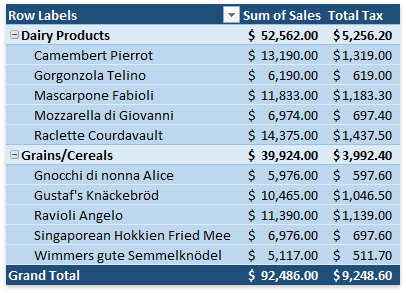.NET Framework 4.5.2+

# How to: Create a Calculated Field

If the predefined aggregation functions or Show Values As calculation options do not meet your requirements, you can create your own formulas to calculate values in a PivotTable report by inserting calculated fields and calculated items.

All calculated fields for a pivot table are stored in the PivotCalculatedFieldCollection collection, which can be accessed using the PivotTable.CalculatedFields property. Use the collection’s methods to create, modify or remove a calculated field.

## Create a Calculated Field

To create a calculated field, use the PivotCalculatedFieldCollection.Add method. The first parameter of this method allows you specify a formula for the calculated field.

A formula string should conform to the common syntax rules and contain only supported elements.

• In the formula, you can use constants and refer to other fields in the PivotTable report. The calculation will be performed on the sum of the underlying data for any fields in the formula. When you reference a field in your formula, you can enclose its name in apostrophes or omit them.
• You cannot create formulas that use a cell reference, defined name, circular references and arrays.
• You cannot use worksheet functions that require cell references or defined names as arguments.
• The formula cannot refer to the PivotTable’s subtotals, totals and Grand Total value.

After the calculated field is created, add it to the PivotTable’s data area using the PivotDataFieldCollection.Add method of the PivotTable.DataFields collection.

Note

Calculated fields are stored in the PivotCache and available to all pivot tables that share the same cache.

The following code demonstrates how to create a field that calculates a 10% sales tax for values in the “Sales” field.

``````Worksheet worksheet = workbook.Worksheets["Report1"];
workbook.Worksheets.ActiveWorksheet = worksheet;

// Access the pivot table by its name in the collection.
PivotTable pivotTable = worksheet.PivotTables["PivotTable1"];
// Create a calculated field based on data in the "Sales" field.
PivotField field = pivotTable.CalculatedFields.Add("=Sales*10%", "Sales Tax");
// Add the calculated field to the data area and specify the custom field name.
PivotDataField dataField = pivotTable.DataFields.Add(field, "Total Tax");
// Specify the number format for the data field.
dataField.NumberFormat = @"_([\$\$-409]* #,##0.00_);_([\$\$-409]* (#,##0.00);_([\$\$-409]* "" - ""??_);_(@_)";
``````

The resulting PivotTable report is shown in the image below (the workbook is opened in Microsoft® Excel®).## Modify a Calculated Field

To change a formula for a calculated field, get access to the required field by its name or index in the PivotCalculatedFieldCollection collection and then assign a new formula to the field’s PivotField.Formula property. To rename a calculated field, use the PivotField.Name property.

``````Worksheet worksheet = workbook.Worksheets["Report1"];
workbook.Worksheets.ActiveWorksheet = worksheet;

// Access the pivot table by its name in the collection.
PivotTable pivotTable = worksheet.PivotTables["PivotTable1"];
// Create a calculated field based on data in the "Sales" field.
// Access the calculated field by its name in the collection.
PivotField field = pivotTable.CalculatedFields["Sales Tax Rate 10"];
//Change the formula for the calculated field.
field.Formula = "=Sales*15%";
//Change the calculated field name.
field.Name = "Sales Tax Rate 15";
//Add the calculated field to the data area and specify the custom field name.
PivotDataField dataField = pivotTable.DataFields.Add(field, "Total Tax");
// Specify the number format for the data field.
dataField.NumberFormat = @"_([\$\$-409]* #,##0.00_);_([\$\$-409]* (#,##0.00);_([\$\$-409]* "" - ""??_);_(@_)";
``````

## Remove a Calculated Field

To remove a particular calculated field from the collection, use the PivotCalculatedFieldCollection.Remove or PivotCalculatedFieldCollection.RemoveAt method. To remove all calculated fields from the collection at once, use the PivotCalculatedFieldCollection.Clear method.

``````Worksheet worksheet = workbook.Worksheets["Report1"];
workbook.Worksheets.ActiveWorksheet = worksheet;

// Access the pivot table by its name in the collection.
PivotTable pivotTable = worksheet.PivotTables["PivotTable1"];
// Create a calculated field based on data in the "Sales" field.